Volume 1, Issue 3, December 2015, Pages: 52-54

Groups of Rectangular Matrices from a Group of Square Matrices

Christian Rakotonirina

Department of Civil Engineering, Institut Supérieur de Technologie d'Antananarivo (IST-T), Antananarivo, Madagascar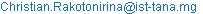Christian Rakotonirina. Groups of Rectangular Matrices from a Group of Square Matrices. Advances in Sciences and Humanities. Vol. 1, No. 3, 2015, pp. 52-54. doi: 10.11648/j.ash.20150103.11

Abstract: Extension of the usual matrix product has been defined in order that two any matrices have product. From a group of square matrices many groups of rectangular matrices for the extended product can be constructed. Then an example of a group of square matrices whose identity element is not the identity matrix has been given.

Keywords: Rectangular Matrices, Usual Product of Matrices, Extended Product of Matrices, Groups

Contents

1. Introduction

Groups theory, especially the groups of square matrices, is among the important mathematics apparatuses use in physics. But, in some papers in theoretical physics [1-4] we notice the use of rectangular matrices. For example,  uses rectangular matrices for constructing the generators of SU(5). That makes us to write in this short communication about groups of rectangular matrices.

A group is a set where, at first an operation is defined. This operation satisfies closure, existence of identity element, each element has an inverse with respect to the identity element and associativity. As the title indicates, we are going to talk the construction of groups of rectangular matrices from a group of square matrices. So it follows that the operation of the group of square matrices of the same dimension should be extended to some sets of rectangular matrices of the same dimension, and then the extended operation will make the sets of rectangular matrices groups. The operation in the groups of square matrices we are going to talk in this paper is the usual multiplication of matrices.

Therefore, in this paper we will define at first the extended multiplication of matrices. Throughout this paper we will use these two products, usual multiplication or usual product and extended multiplication or extended product. Some propositions will be given, according to which we will be able to construct groups of rectangular matrices for the extended product, from a group of square matrices for the usual product. Finally, we will show that the groups of rectangular matrices constructed for this way, will be able to be talked in terms of groups of square matrices for the usual product.

2. Extended Product of Matrices

In the following definition we are going to extend the usual product of a matrixby a matrix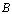, with the number of the columns ofis equal to the number of the rows of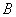, to the product of any two matrices.

Definition 1 Let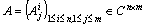,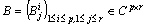.

Define the product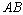of the matrix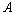by the matrix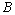as the matrix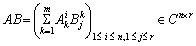if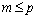, and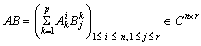if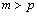.

If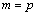the extended product is not other than the usual product.

Example 2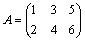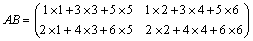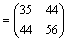This is like if we did the product of the square matrix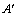by the matrix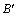obtained respectively in adding zeros at the right side or underside of the rectangular matricesand.,Then after we have done the usual product we delete the zeros at the sides.

Thus, it is obvious that the extended multiplication is associative.

3. Constructing Groups of Rectangular Matrices

According to the definition of the multiplication above, from any group of square matrices of the same dimension many groups of rectangular matrices of the same dimension can be constructed, in adding zeros at the right side or underside of the matrices.

Example 3 Consider the group S2 of the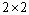permutation matrices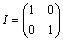,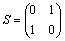The set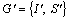of rectangular matrices with, for example,,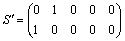is a group for the extended multiplication.

Whereas, the set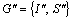of square matrices, with,is a group for the usual multiplication.

In the propositions below the identity element of the group is not necessarily the identity matrix.

For anymatrix, for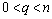, denote by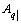the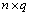matrix, extracted fromin deleting the latest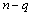columns of. Denote by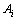the column matrix equal to the i-th column of.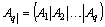.

Proposition 4 Let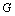be a group ofmatrices, for the usual multiplication. For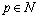,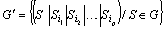is a group of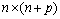matrices, for the extended multiplication.

Example 5 Let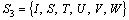be the group of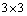permutation matrices.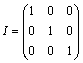,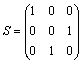,,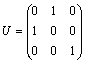,,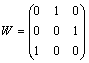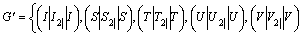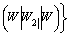is a group of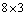matrices for the extended multiplication, where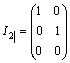,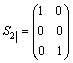,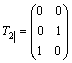,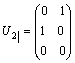,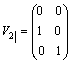,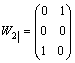Counter-example 6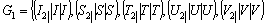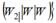is not a group for the extended multiplication, because it has not identity element.

Example 7 Consider the group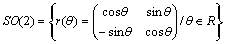of the rotation in a plane, for the usual multiplication. Then, the setis a group of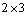matrices for the extended multiplication.

For anymatrix, for, denote by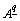the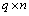matrix, extracted fromin deleting the latestrows of. Denote bythe row matrix equal to the i-th row of.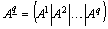.

Proposition 8 Letbe a group ofmatrices, for the usual multiplication. For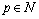,is a group of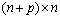matrices, for the extended multiplication.

For a group of rectangular matrices for the extended multiplication, in adding zeros at the right side or underside for each rectangular matrix, element of this group, until we obtain a set of square matrices of the same dimension, this last set is a group of square matrices for the usual multiplication.

Example 9 Consider the group of thematrices of the example 7.We add zeros at the underside of the rectangular matrices of this group, until they become square matrices. Then, the set of square matricesobtained is a group for the usual multiplication.

According to the proposition 8 the set of rectangular matricesis a group for the extended product and the set of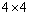matricesis a group for the usual product, whose identity element is4. Conclusion and Discussion

The extended product will not be other than the usual product if we consider a rectangular matrix as square matrix after adding zeros at the underside or right side. We think that there is no danger if we replace the usual product by the extended product because the usual product is particular case of the extended product. Then we can do the product of anyone matrix by any other matrix.

From a group of square matrices of the same dimension, for the usual product, we can construct many groups of rectangular matrices for the extended product. In the case of finite groups, the group of the square matrices and the groups of the rectangular matrices are of the same order.

References

1. Pushpa, P. S. Bisht, Tianjun Li, O. P. S. Negi, "Quaternion Octonion Reformulation of Grand Unified Theories", International Journal of Theoretical Physics., 51(10), (2012), 3228-3235.
2. D. Berenstein, "A Matrix Model for a Quantum Hall Droplet with manifest Particle-hole Symmetry", Phys. Rev. D71, 085001,(2005).
3. M. Srednicki, "A new Construction of the Penner Model", Mod. Phys.Lett.A07,2857-2860,(1992), DOI:10.1142/S0217732392 004237.
4. S. Majid, "On the Addition of Quantum Matrices", Journal of Mathematical Physics 08, (1993), DOI:10.1063/1.530527.

 Contents 1. 2. 3. 4.
Article ToolsAbstractPDF(159K)